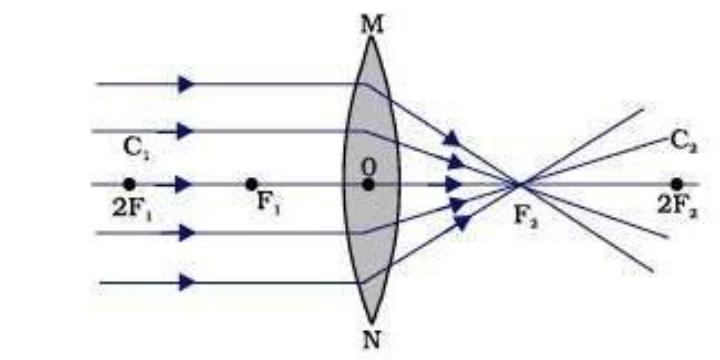## Refraction through spherical lenses

Lens: A piece of transparent material (such as glass) whose two opposite regular surfaces are either curved or one is curved and the other is flat and which is used individually or in combination to create an image that focuses light rays.

● The history behind the lens: It is generally accepted that the origin of the lens is traced back to the ancient Roman philosopher Seneca, who described about 2,000 years ago that “letters can be magnified by a crystal ball”. The lens works using a principle known as “refraction” of light: the light bends and changes its direction of travel.

■ What is refraction?

When a ray of light in the air falls on the surface of a transparent medium(like glass), some of it is reflected, when the residue is sent.The larger the angle of incidence, the greater the amount of reflected light.

No medium is completely transparent and some light absorption always occurs, the energy is converted into heat.

This subsequent effect will be ignored. If the direction of the ray inside the medium is not different from the direction of the incident ray .The incident ray is normal (perpendicular) to the surface.The transmitted ray is bent because it crosses the intermediate surface one medium to another. This change of direction of the ray on the surface is called refraction.

□ Refracting material: Common refracting materials are glass (high-grade optical quality glass) and various types of plastics. These have been chosen not only for the side effects of the light rays passing through them, but also for them transparency, uniformity and their resistance against atmospheric corrosion.

Optical glass is commonly used manufacture of prisms and lenses. There are two main types of optical glass available: Crown and Flint. The former Silica (sand, SiO2) and salt compounds of sodium and potassium. In addition, small amounts of other materials Such as barium and zinc oxide. Flint glasses, in addition to the above components, contain oxides Lead and crowns thicker than glasses. Some plastic materials are now increasingly being used for eye contact lenses.

In this category, the  thermosetting material allyl diglycol carbonate, commonly known as CR-39 (the CR standing for Columbia Resin). These plastics start as linear polymer chains that get cross-linked permanently during molding.Therefore they cannot be remolded.

■ Here, spherical lenses in the form of plano-convex, double convex and plano-concave. We call both plano convex and double convex lenses positive lenses; They have a positive focal length. Plano convex lenses are round on one surface and flat on the other.

These are often chosen when one works with infinite or near-infinite conjugates to apply a combination of light and monochromatic illumination. Their special shape reduces spherical distortion in a ratio of approximately 5: 1 and they are often the most practical choice for on-demand applications. In a standard setup the curved surface will be infinitely connected or face the distance of the largest object.

□ Refraction of round surface

Consider refraction in a circular interface between two transparent media. An infinite portion of a spherical surface can be considered as a planar and the same rule of refraction can be applied to each point on the surface.

The incident rays extend from one medium of refractive index n1 to another m,edium of refractive index n2.

It is assumed that the surface holes (or lateral shapes) will be smaller than the other distances involved, so that smaller angles can be estimated.

■ Refraction of round surface

Consider refraction in a circular interface between two transparent media. An infinite portion of a spherical surface can be considered as a planar and the same rule of refraction can be applied to each point on the surface.

The incident rays extend from one medium of refractive index n1 to another m,edium of refractive index n2.

It is assumed that the surface holes (or lateral shapes) will be smaller than the other distances involved, so that smaller angles can be estimated.

Consider that NM is taken as approximately equal to the length of the perpendicular from the point N on the principal axis.

Considering small angles,

tan<NOM = (MN)/(OM)

tan < NCM = (MN/MC)

tan<NIM = (MN)/(MI)

Now, for ΔNOC, <i is the exterior angle. Therefore, i = ∠NOM + ∠NCM

i = (MN/OM)+(MN/MC) (equation (1))

Similarly, r = ∠NCM – ∠NIM

e., r = (MN/MC) – (MN/MI) (equation (2))

Now, by Snell’s law n1 sin i = n2 sin r or for small angles

n1i = n2r

Substituting i and r from Equation. (1) and (2), we get

(n1/OM) + (n2/MI) = (n2 – n1)/MC) Equation (3)

Here, OM, MI and MC represent magnitudes of distances. Applying the Cartesian sign convention,

OM = –u, MI = +v, MC = +R

Substituting these in Eq. (3),

we get,

(n2 –v) –(n1/u) = (n2 – n1)/(R)  Equation (4)

Equation (4) gives us a relation between object and image distance in terms of refractive index of the medium and the radius of curvature of the curved spherical surface. It holds for any curved spherical surface.

### Sagata Saha

The secret of success is to do the common thing uncommonly well.